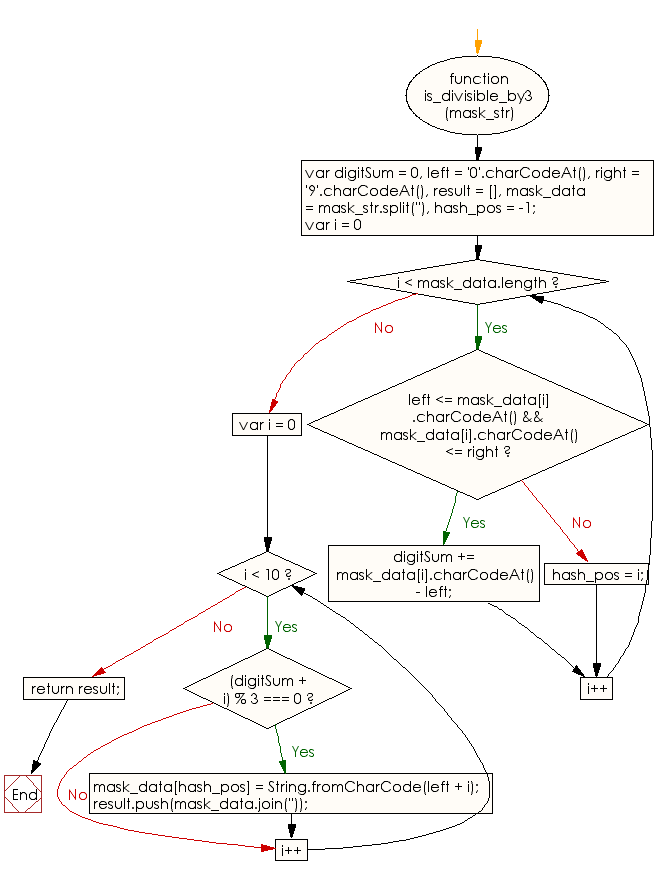# JavaScript: Find all the possible options to replace the hash in a string

## JavaScript Basic: Exercise-116 with Solution

Write a JavaScript program to find all the possible options to replace the hash in a string (Consists of digits and one hash (#)) with a digit to produce an integer divisible by 3.

For a string "2*0", the output should be : ["210", "240", "270"]

Sample Solution:

HTML Code:

``````<!DOCTYPE html>
<html>
<meta charset="utf-8">
<meta name="viewport" content="width=device-width">
<title> Find all the possible options to replace the hash in a string</title>
<body>

</body>
</html>
```
```

JavaScript Code:

``````function is_divisible_by3(mask_str) {

var digitSum = 0,
left = '0'.charCodeAt(),
right = '9'.charCodeAt(),
result = [],
hash_pos = -1;

for (var i = 0; i < mask_data.length; i++) {
{
}
else
{
hash_pos = i;
}
}

for (var i = 0; i < 10; i++) {
if ((digitSum + i) % 3 === 0) {
}
}

return result;
}

console.log(is_divisible_by3("2#0"))
console.log(is_divisible_by3("4#2"))
``````

Sample Output:

```["210","240","270"]
["402","432","462","492"]
```

Flowchart:ES6 Version:

``````function is_divisible_by3(mask_str) {
let digitSum = 0;
const left = '0'.charCodeAt();
const right = '9'.charCodeAt();
const result = [];
let hash_pos = -1;

for (var i = 0; i < mask_data.length; i++) {
{
}
else
{
hash_pos = i;
}
}

for (var i = 0; i < 10; i++) {
if ((digitSum + i) % 3 === 0) {
}
}

return result;
}

console.log(is_divisible_by3("2#0"))
console.log(is_divisible_by3("4#2"))
``````

Live Demo:

See the Pen javascript-basic-exercise-116 by w3resource (@w3resource) on CodePen.

Improve this sample solution and post your code through Disqus

What is the difficulty level of this exercise?

﻿

## JavaScript: Tips of the Day

Promise object

```const myPromise = () => Promise.resolve('I have resolved!');

function firstFunction() {
myPromise().then(res => console.log(res));
console.log('second');
}

async function secondFunction() {
console.log(await myPromise());
console.log('second');
}

firstFunction();
secondFunction();
```

With a promise, we basically say I want to execute this function, but I'll put it aside for now while it's running since this might take a while. Only when a certain value is resolved (or rejected), and when the call stack is empty, I want to use this value.
We can get this value with both .then and the await keyword in an async function. Although we can get a promise's value with both .then and await, they work a bit differently.
In the firstFunction, we (sort of) put the myPromise function aside while it was running, but continued running the other code, which is console.log('second') in this case. Then, the function resolved with the string I have resolved, which then got logged after it saw that the callstack was empty.
With the await keyword in secondFunction, we literally pause the execution of an async function until the value has been resolved before moving to the next line.
This means that it waited for the myPromise to resolve with the value I have resolved, and only once that happened, we moved to the next line: second got logged.

Ref: https://bit.ly/3jFRBje